# Industry News Display

2014-04-15   Control Engineering

## Understand the basics to drive greater efficiency.

By: Bill Gatheridge, Yokogawa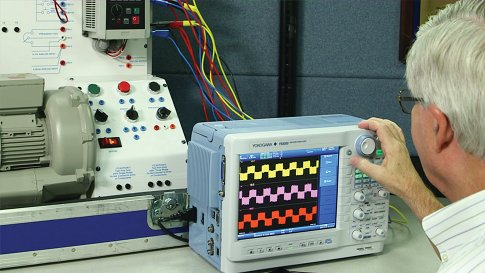Energy is one of the highest cost items in a plant or facility, and motors often consume the lion's share of plant power, so making sure motors are operating optimally is vital. Accurate power measurements can help to reduce energy consumption, as measurement is always the first step toward better performance and can also help extend the life of a motor. Small misalignment or other issues are often invisible to the naked eye, and the slightest wobble in a shaft can negatively affect productivity and quality, and even shorten the life of the motor.

Over the next three issues of Applied Automation, we will discuss a three-step process for making precision electrical and mechanical power measurements on a variety of motors and variable speed drive (VFD) systems. We will also show how these measurements are used to calculate the energy efficiency for motor and drive systems.

In addition, we will provide an understanding of how to make precision power measurements on complex distorted waveforms, as well as what instruments to use for different applications.

Basic electrical power measurements

Electric motors are electromechanical machines that convert electric energy into mechanical energy. Despite differences in size and type, all electric motors work in much the same way: an electric current flowing through a wire coil in a magnetic field creates a force that rotates the coil, thus creating torque.

Understanding power generation, power loss, and the different types of power measured can be intimidating, so let's start with an overview of basic electric and mechanical power measurements.

What is power? In the most basic form, power is work performed over a specific amount of time. In a motor, power is delivered to the load by converting electrical energy per the following laws of science.

In electrical systems, voltage is the force required to move electrons. Current is the rate of the flow of charge per second through a material to which a specific voltage is applied. By taking the voltage and multiplying it by the associated current, the power can be determined.

P = V * I where power (P) is in watts, voltage (V) is in volts, and current (I) is in amperes

A watt (W) is a unit of power defined as one Joule per second. For a dc source the calculation is simply the voltage times the current: W = V x A. However, determining the power in watts for an ac source must include the power factor (PF), so:
W = V x A x PF for ac systems.

The power factor is a unitless ratio ranging from -1 to 1, and represents the amount of real power performing work at a load. For power factors less than unity, which is almost always the case, there will be losses in real power. This is because the voltage and current of an ac circuit are sinusoidal in nature, with the amplitude of the current and voltage of an ac circuit constantly shifting and not typically in perfect alignment.

Since power is voltage times current (P = V*I), power is highest when the voltage and current are lined up together so that the peaks and zero points on the voltage and current waveforms occur at the same time. This would be typical of a simple resistive load. In this situation, the two waveforms are "in phase" with one another and the power factor would be 1. This is a rare case, as almost all loads aren't simply and perfectly resistive.

Two waveforms are said to be "out of phase" or "phase shifted" when the two signals do not correlate from point to point. This can be caused by inductive or nonlinear loads. In this situation, the power factor would be less than 1, and less real power would be realized.

Due to the possible fluctuations in the current and the voltage in ac circuits, power is measured is a few different ways.

Real or true power is the actual amount of power being used in a circuit, and it's measured in watts. Digital power analyzers use techniques to digitize the incoming voltage and current waveforms to calculate true power, following the method in Figure 2: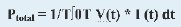Figure 2: True power calculation

In this example the instantaneous voltage is multiplied by the instantaneous current (I) and then integrated over a specific time period (t). A true power calculation will work on any type of waveform regardless of the power factor (Figure 3).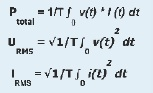Figure 3: These equations are used to calculate a true power measurement and true RMS measurements.

Harmonics create an additional complication. Even though the power grid nominally operates at a frequency of 60Hz, there are many other frequencies or harmonics that potentially exist in a circuit, and there can also be a dc or direct current component. Total power is calculated by considering and summing all content, including harmonics.

The calculation methods in Figure 3 are used to provide a true power measurement and true RMS measurements on any type of waveform, including all harmonic content, up to the bandwidth of the instrument.

Power measurement

We'll next look at how to actually measure watts in a given circuit. A wattmeter is an instrument that uses voltage and current to determine power in watts. The Blondel Theory states that total power is measured with a minimum of one fewer wattmeter than the number of wires. For example, a single-phase two-wire circuit will use one wattmeter with one voltage and one current measurement.

A single-phase three-wire split-phase system is often found in common housing wiring. These systems require two wattmeters for power measurement.

Most industrial motors use three-phase three-wire circuits that are measured using two wattmeters. In the same fashion, three wattmeters would be necessary for a three-phase four-wire circuit, with the fourth wire being the neutral.

Figure 4 shows a three-phase three-wire system with load attached using the two-wattmeter method for measurement. Two line-to-line voltages and two associated phase currents are measured (using wattmeters Wa and Wc). The four measurements (line-to-line and phase current and voltage) are utilized to achieve the total measurement.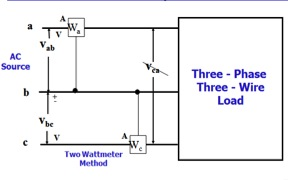Figure 4: Measuring power in a three-phase three-wire system with two wattmeters.

Since this method requires monitoring only two current and two potential transformers instead of three, installation and wiring configuration are simplified. It can also measure power accurately on a balanced or an unbalanced system. Its flexibility and low-cost installation make it a good fit for production testing in which only the power or a few other parameters need measurement. For engineering and research and development work, the three-phase three-wire with three-wattmeter method is best as it provides additional information that can be used to balance loading and determine true power factor. This method uses all three voltages and all three-currents. All three voltages are measured (a to b, b to c, c to a), and all three-currents are monitored.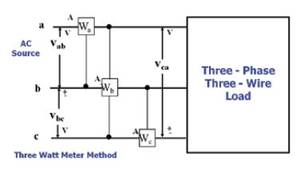Figure 5: When designing motors and drives, seeing all three voltages and currents is key, making the three-wattmeter method in the figure above the best choice.

Power factor measurement

In determining the power factor for sine waves, the power factor is equal to the cosine of the angle between the voltage and current (Cos Ø). This is defined as the "displacement" power factor, and is correct for sine waves only. For all other waveforms (non-sine waves), the power factor is defined as real power in watts divided by apparent power in voltage-amperes. This is called the "true" power factor and can be used for all waveforms, both sinusoidal and non-sinusoidal.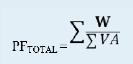Figure 6: Total power factor is determined by summing the total watts divided by the total VA measurement.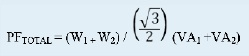Figure 7: Using the two-wattmeter method, the sum of the total watts (W1 + W2) is divided by the VA measurements.

However, if the load is unbalanced (the phase currents are different), this could introduce an error in calculating the power factor because only two VA measurements are used in the calculation. The two VAs are averaged because it's assumed they're equal; however, if they're not, a faulty result is obtained.

Therefore, it's best to use the three-wattmeter method for unbalanced loads because it will provide a correct power factor calculation for either balanced or unbalanced loads.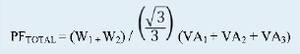Figure 8: With the three-wattmeter method, all three VA measurements are used in the above power factor calculation.

Power analyzers use the method above, which is called the 3V-3A (three-voltage three-current) wiring method. This is the best method for engineering and design work because it will provide a correct total power factor and VA measurements for a balanced or unbalanced three-wire system.

Basic mechanical power measurements

In an electric motor, the mechanical power is defined as the speed times the torque. Mechanical power is typically defined as kilowatts or horsepower, with one W equaling 1 Joule/sec or 1 Nm/sec.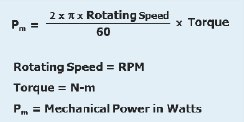Figure 9: Mechanical power measurements in watts are defined as two times Pi times the rotating speed (rpm) divided by 60 times the torque (Newton-Meter).

Horsepower is the work done per unit of time. One hp equals 33,000 pound feet per minute. Converting hp to watts is achieved using this relationship: 1 hp = 745.69987 W. However, the conversion is often simplified by using 746W per hp (Figure 10).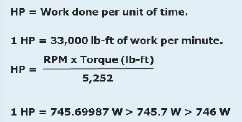Figure 10: Mechanical power measurement equations for horsepower often use a rounded figure of 1 hp = 746W.

For ac induction motors, the actual or rotor speed is the speed at which the shaft (rotor) rotates, typically measured using a tachometer. The synchronous speed is the speed of the stator's magnetic field rotation, calculated as 120 times the line frequency divided by the number of poles in the motor. Synchronous speed is the motor's theoretical maximum speed, but the rotor will always turn at a slightly slower rate than the synchronous speed due to losses, and this speed difference is defined as slip.

Slip is the difference in the speed of the rotor and the synchronous speed. To determine the percentage of slip, a simple percentage calculation of the synchronous speed minus the rotor speed divided by the synchronous speed is used.

Efficiency can be expressed in simplest form as the ratio of the output power to the total input power or efficiency = output power/input power. For an electrically driven motor, the output power is mechanical while the input power is electrical, so the efficiency equation becomes efficiency = mechanical power/electrical input power.

Bill Gatheridge is the product manager for power measuring instruments at Yokogawa Test & Measurement, and has more than 20 years of experience with the company in the area of precision electrical power measurements. He is a member and vice chairman of the ASME PTC19.6 committee on electrical power measurements for utility power plant performance testing.

This article appears in the Applied Automation supplement for Control Engineering and Plant Engineering